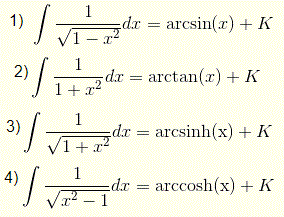### Integral

Integration can be used to find areas, volumes, central points and many useful things. But it is often used to find the area under the graph of a function.

The process of finding a function, given its derivative, is called anti-differentiation (or integration). If F'(x) = f(x), we say F(x) is an anti-derivative of f(x).

Integration is the reverse of differentiation

### Indefinite Integral

A Indefinite Integral has no start and end values. In other word, there is no limit.

ddxF(x) = f(x) ⟺ ∫f(x)dx = F(x) + C

This is called indefinite integration,### Definite Integral

A Definite Integral has start and end values.
The definite integral given by a function f of a real variable x and an interval [a, b] of the real line.

a
∫ f(x) dx = [F(x)]ba = F(b)-F(a)
b

And replace b by a, we should have the answer to be 0 because the area under the curve from b = a to b = a, should obviously be 0.

Some​​ of​​ the​​ important​​ integration​​ formulas​​ are​​ listed​​ below​​ :-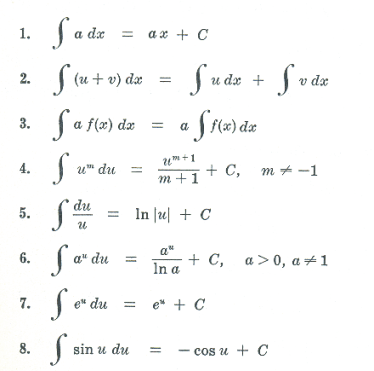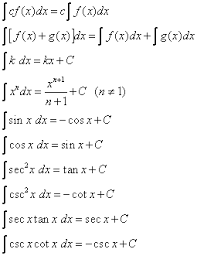​​ ​​ ​​ ​​ ​​ ​​ ​​ ​​ ​​ ​​ ​​ ​​ ​​ ​​ ​​ ​​ ​​ ​​ ​​ ​​ ​​ ​​ ​​ ​​ ​​ ​​ ​​ ​​ ​​ ​​ ​​ ​​ ​​ ​​ ​​​​ Fig.(a) ​​ ​​ ​​ ​​ ​​ ​​ ​​ ​​ ​​ ​​ ​​ ​​ ​​ ​​ ​​ ​​ ​​ ​​ ​​ ​​ ​​ ​​ ​​ ​​ ​​ ​​ ​​ ​​ ​​ ​​ ​​ ​​ ​​ ​​ ​​ ​​ ​​ ​​ ​​ ​​ ​​ ​​ ​​ ​​ ​​ ​​ ​​ ​​ ​​ ​​ ​​ ​​ ​​ ​​ ​​ ​​ ​​ ​​ ​​ ​​ ​​ ​​ ​​ ​​ ​​ ​​ ​​ ​​ ​​ ​​ ​​ ​​ ​​ ​​ ​​ ​​ ​​ ​​ ​​ ​​ ​​ ​​ ​​ ​​ ​​ ​​ ​​ ​​ ​​​​ fig.(b)

1.For​​ an​​ example​​ ,find​​ the​​ integration​​ of​​

sin 4 x

.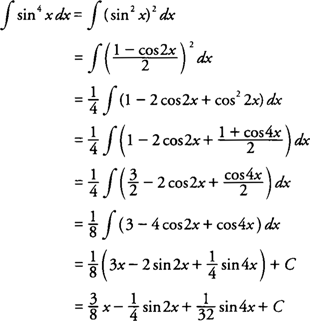Some​​ other​​ important​​ formulas​​ given​​ by.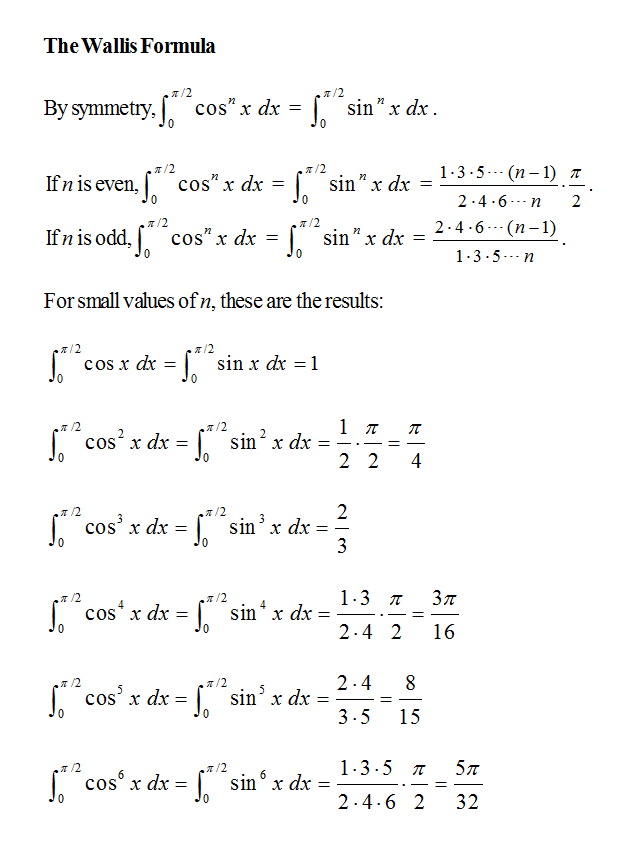Some​​ inverse​​ formulas.